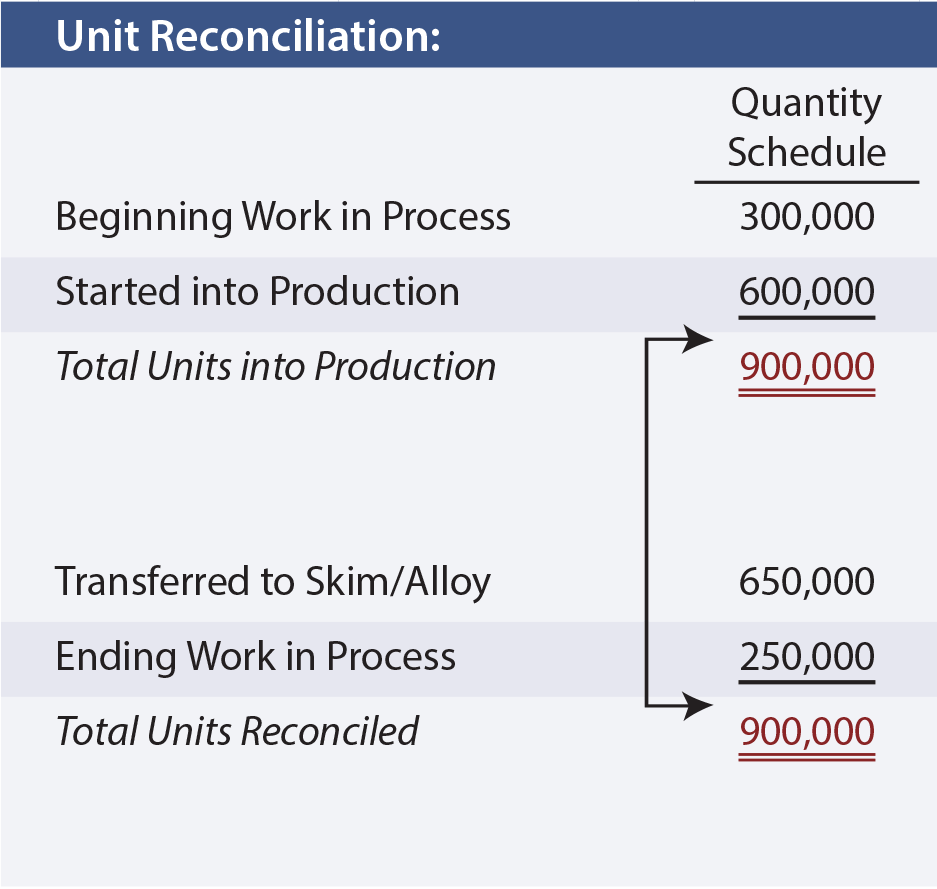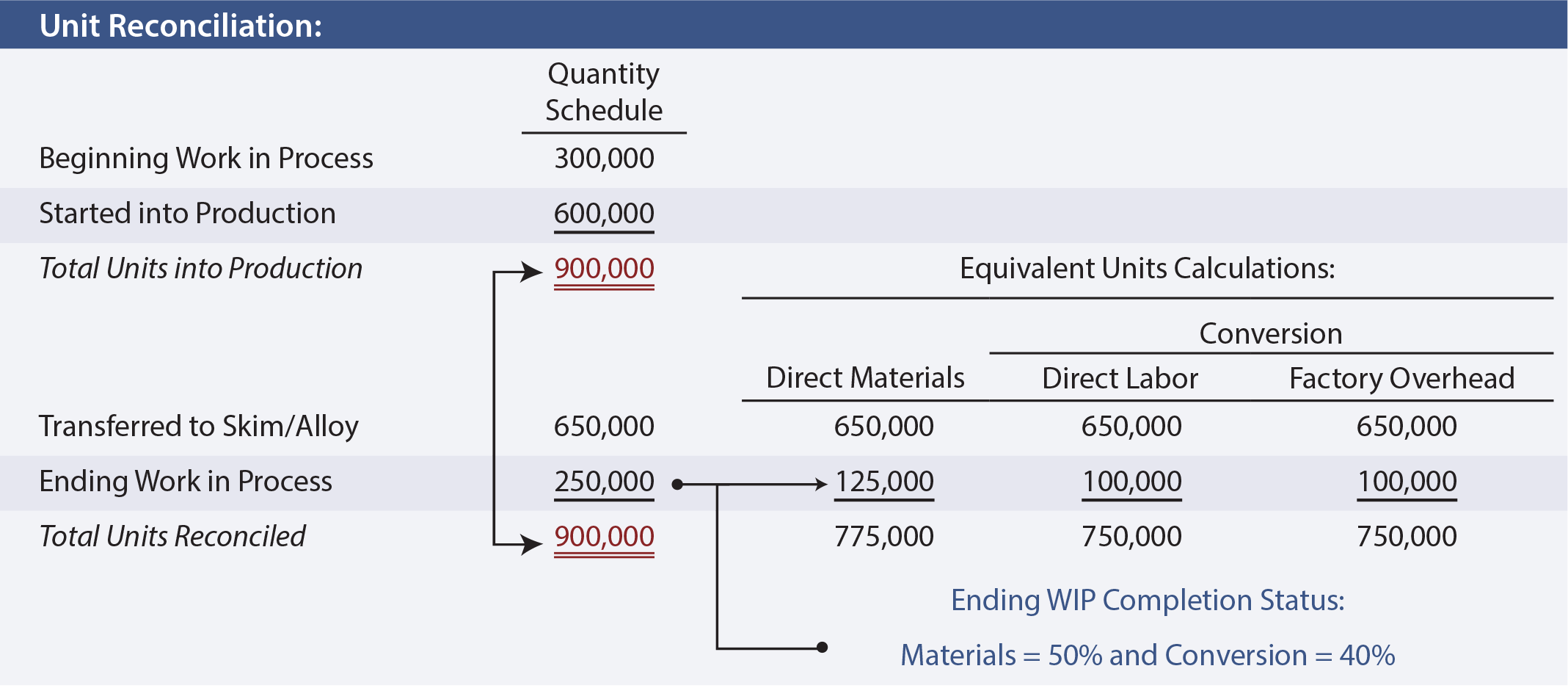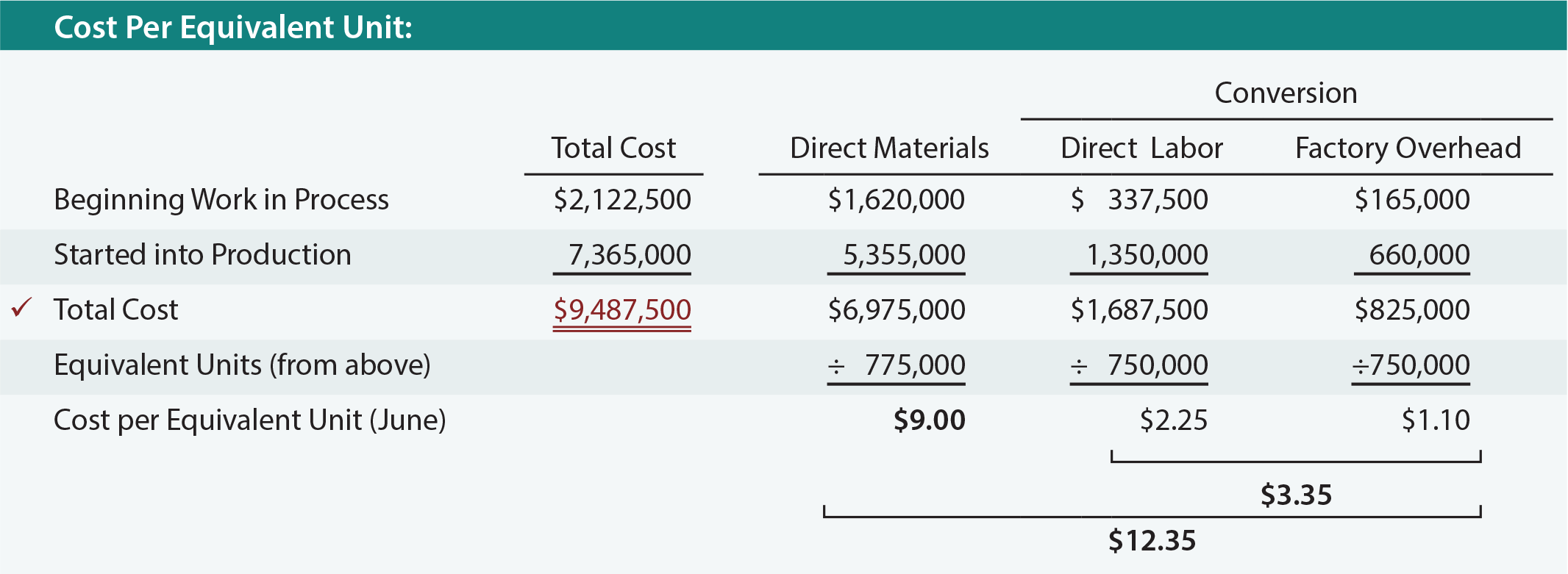When goods are produced in a continuous process, how are costs to be allocated between work in process and finished goods? Accountants have devised the concept of an equivalent unit, a physical unit expressed in terms of a finished unit.

For example, ten units in process that are 30% complete equate to three equivalent units of output. None of the ten units is complete; merely the equivalent amount of work necessary to complete three units is said to have been performed.

## Factors Of Production

Equivalent units must be considered relative to each of the factors of production. In other words, 80% of necessary direct material may be in process but only 60% of the direct labor and factory overhead. Therefore, proper costing methodology for 100 units in process would entail 80 equivalent units of material, and 60 equivalent units of conversion (i.e., labor and overhead).

To assess the equivalent units of production requires careful reasoning about the amount of direct material injected into production for each department, relative to the total amount of direct material that will ultimately be needed to complete the process within that department. This type of assessment must be repeated for direct labor and overhead. If overhead is applied based on labor, the process is simplified because the “percent complete” would be the same for labor and overhead.

However, if overhead were applied on some other basis (like machine hours) then a separate determination of equivalency would be needed for labor and for overhead (the illustrations within this chapter will assume overhead is applied based on labor).

## ExampleTo better understand equivalent units, focus on an example for Navarro Steel. To begin, one needs to identify the total units that are to be considered, no matter their stage of completion at the beginning and end of the month.

The first stage in Navarro’s production process is the Melting Department. Navarro started the month of June with 300,000 tons of iron ore in process in the Melting Department. During June, an additional 600,000 tons were introduced into the melting vats.

This means 900,000 total units must be reconciled. The quantity schedule provides this reconciliation. It shows that 650,000 units were transferred on to the Skim/Alloy Department, leaving 250,000 tons still in process.

In reconciling total units into production with the total units transferred out/still in process, it is not uncommon for there to be a shortfall. The reason is that many processes may involve scrap, waste, or spoilage (e.g., evaporation, spilling, etc.). Waste and spoilage would be added as a third component needed to balance the lower portion of the quantity schedule column.

Advanced managerial accounting courses usually demonstrate the mechanics of handling the cost of spoilage within a process cost system. However, for now simply know that the cost of “normal” spoilage should be allocated to the inventory of good units, while the cost of excessive waste is charged off as a loss on the income statement.

Once the total units have been reconciled, the equivalent units are computed. The correct manipulation of the data will depend on the inventory method in use. In this illustration, Navarro is assumed to use the weighted-average costing method (other approaches such as FIFO could be used). This simplifies the process because the beginning inventory and current period production can be combined or “averaged” together.

Thus, the 650,000 units that were completed are counted as 650,000 equivalent units of output no matter their physical origin from beginning inventory or otherwise. However, the units in ending work in process require more thoughtful consideration.

Assume that the 250,000 tons in ending work in process are 50% complete with respect to material (i.e., 125,000 equivalent units) and 40% complete with respect to conversion (i.e., 100,000 equivalent units). The following schedule shows how the total units are translated into equivalent units:## Cost Per Equivalent Unit

The equivalent unit calculations are carried forward into the “cost per equivalent unit” schedule. This shows how the combined costs from beginning work in process (assumed at \$2,122,500) and current period production (assumed at \$7,365,000) are divided by the equivalent units. The result is the weighted-average cost per equivalent unit for each factor of production. The individual cost factors can be combined to identify conversion cost and overall cost per equivalent unit.Did you learn?
What is an equivalent unit of production and what are conversion costs?
Why is it important to differentiate between materials and conversion costs?
Calculate equivalent units of production, taking into account beginning work in process, and current period production amounts (using the weighted-average method).